# 30

## Summary

### Factorization

The factorization is as follows, with factors 2, 3, and 5: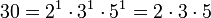### Properties and families

Property or family Parameter values First few members of the family Proof of satisfaction/membership/containment
Giuga number: composite numbersuch thatdividesfor all prime divisorsof. first Giuga number 30, 858, 1722, 66198, [SHOW MORE]View list on OEIS check on each prime divisor:
2 divides (30/2) - 1 = 14
3 divides (30/3) - 1 = 9
5 divides 30/5 - 1 = 5
primorial: product of the first few prime numbers product of the first three prime numbers (if we start the indexing from the zeroth primorial, which is an empty product and evaluates to 1, it is the fourth in the list) 1, 2, 6, 30, 210, 2310 View list on OEIS The first three primes are 2,3,5, and their product is 30.

## Arithmetic functions

Function Value Explanation
Euler totient function 8 The Euler totient function is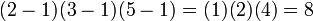.
universal exponent 4 The universal exponent is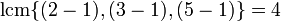.
divisor count function 8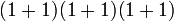where the first 1s in both factors are the multiplicities of the prime divisors.
divisor sum function 72times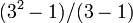times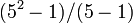equals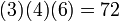.
Mobius function -1 The number is square-free and has an odd number of prime divisors (3 prime divisors).